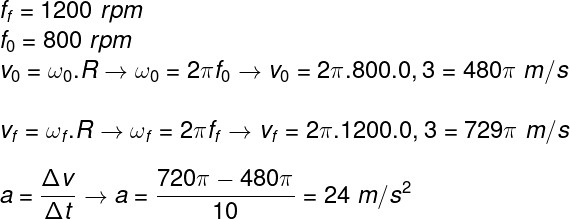Mechanics

# Uniformly varied circular motion

Uniformly Varied Circular Motion (MCUV) is one in which a body follows a circular path of constant radius with variable angular velocity. In addition to presenting centripetal acceleration, in the MCUV there is angular acceleration (α).

## angular velocity

Before looking at the MCUV, we need to remember a little about uniform circular motion (MCU) , that is, motion along a circular path that occurs with constant angular velocity .

It is worth remembering that, in the MCUV, the angular velocity is variable . This indicates that, in a particle that moves in MCUV, the vector that connects it to the center of the trajectory travels through different angles at each time interval . When a particle in MCUV exhibits a positive acceleration, the time required for it to complete one revolution decreases, otherwise it increases.

Let us consider a particle that, at the initial instant t 0 , is positioned at the initial angle φ 0 , as shown in the figure:

After a certain time interval, at time t f , the particle has moved and is in position φ F :

By subtracting the final angle from the initial angle, we get the angular displacement (Δφ = φ F – φ 0 ). The angular velocity, in turn, is calculated by the ratio between the angular displacement and the time interval.

However, this equation is valid for a body that describes a uniform circular motion , in the case of the MCUV, it is the angular acceleration of the particle, so the angular velocity for this motion is calculated as follows: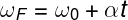ω F and ω 0 – final and initial angular velocity (rad/s)

t – time interval

Where α represents the angular acceleration, and t, the time interval. The variables ω F and ω 0 are, respectively, the final and initial angular velocities, whose unit of measure is rad/s. The unit of measurement for angular acceleration is rad/s² .

## Tangential, centripetal, angular and resultant acceleration

In uniformly varied circular motion, there are several accelerations, so it is important to know each of them. Let’s start with the angular acceleration, which is equal to the change in angular velocity during a certain time interval:Then we have the scalar or tangential acceleration. This acceleration represents the change in speed as a function of time. It is the one that points in the direction tangential to the circular path, and the formula used to calculate it is this: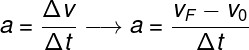F ev 0 – final and initial speeds (m/s)

a – scalar acceleration (m/s²)

Centripetal acceleration is that which is present in all curvilinear motion . It always points to the center of the curve and can be calculated according to the following formula: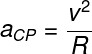CP – centripetal acceleration (m/s²)

v – speed (m/s)

R – radius of the curve (m)

Finally, the resulting acceleration is given by the vector resultant of the scalar (tangential) and centripetal accelerations , since the two are perpendicular to each other. To obtain the direction of the resulting acceleration, we simply apply the parallelogram rule ; to calculate its modulus we use the Pythagorean theorem , note: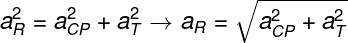## Formulas for uniformly varied circular motion

The formulas for uniformly varied circular motion can be easily written if we remember the main formulas for uniformly varied motion (MUV). It is worth remembering that the equations shown below are related to angular quantities (angular displacement, angular velocity and angular acceleration).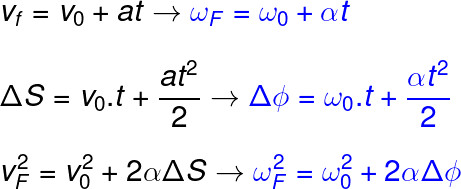In addition to these equations, there are those that relate spatial quantities (position, velocity and acceleration) to angular quantities. In all, there are three equations, check it out: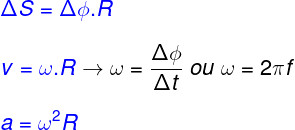## Solved exercises on MCUV

Question 1) A particle moves in a circular path with a constant radius of 0.5 m. It is known that the angular velocity of the particle, at the initial instant of time, was 2.0 rad/s and that, after 4 s, it became 4.0 rad/s. The angular acceleration of this particle is equal to:

Template: Letter A

Resolution:

Let’s use the angular acceleration formula to determine it, check it out: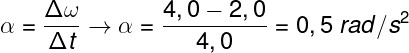Question 2) A washing machine has two speed options during the spin process: 800 rpm and 1200 rpm. Knowing that the machine takes 10.0 s to change speed and that its drum has a radius of 30 cm, the scalar acceleration that the engine of the machine produces between these 10.0 s is equal to:

a) 10 m/s²

b) 12 m/s²

c) 24 m/s²

d) 8 m/s²

e) 4 m/s²

Template: Letter C

Resolution:

Initially it is necessary to discover the magnitude of the initial and final angular velocities. Then we calculate the magnitude of the scalar speeds of the machine at the two operating frequencies and then we calculate the scalar acceleration by dividing the final and initial scalar speeds by the time interval, check: